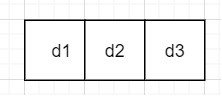# Count positive integers with 0 as a digit and maximum ÔÇÿd' digits in C++

We are given a number d which represents the number of digits. The goal is to find the count of positive integers with 0 as a digit and have maximum d digits. Count all 1 digit, 2 digit, 3 digit….d digit positive numbers containing at least one 0.

We will first find numbers the count of numbers that have d digits with at least one 0. Let’s say d=3. To make a 3-digit number with at least one 0, possible ways are −Here d1 can have 1 to 9 : 9 ways
d2 can have 0-9 : 10 ways
d3 can have 0-9 : 10 ways
Total numbers possible: 9 x 10 x 10 = 9 x 102
For d digits, count of numbers: 9 x 10d-1
For d digits, numbers without any 0 are : 9d
Total numbers having d digits with at least one 0 = 9 x 10d-1 - 9d = 9 x ( 10d-1 - 9d-1 )

Let us understand with examples

Input − d=4

Output − Count of positive integers with 0 as a digit and maximum 'd' digits are − 2619

Explanation − x digit numbers with at least one 0 −

1 digit numbers : 0
2 digit numbers : 9
3 digit numbers : 171
4 digit numbers: 2439
Total= 9+171+2439 = 2619

Input − d=1

Output − Count of positive integers with 0 as a digit and maximum 'd' digits are − 0

Explanation − 1 to 9 no number has 0 as a digit.

## The approach used in the below program is as follows

We will use two approaches. A first naive approach using a for a loop. Start traversing from 1 digit upto d digits and calculate numbers using the formula mentioned above. Add returned value to count.

• Take the integer d for digits.

• Function total_count(int d)) takes a number of digits d and returns a count of numbers with d digits having at least one 0.

• Calculate such numbers as temp=9*(pow(10,d-1) - pow(9,d-1));

• Return temp.

• Function maximum_d(int d) takes the maximum number of digits d and returns count of numbers upto d digits having at least one 0.

• Traverse using a loop starting from 1 digit numbers then 2 and so on till d.

• For each d calculate numbers as total_count(i). Add this to count.

• At last we will get the total count.

• Return count as result.

## Efficient Approach

In this approach, we will calculate the count by observing the G.P formed for the above calculations.

Solution is 9 x (10d-1 - 9d-1)
= 9 x (10d - 1)- 9 x (9d-1)
= 9 x (10i - 1) - 9 x (9i - 1) ( 1<=i<=d )
= g.p 1 - g.p 2
= 9x(10d-1)/(10-1) - 9x(9d-1)/(9-1)
= (10d-1)- (9/8)*(9d-1)
• Take d as the maximum number of digits.

• Function maximum_d(int d) takes the maximum number of digits d and returns a count of numbers up to d digits having at least one 0.

• Using above formula calculate temp_1 as 9*((pow(10,d)-1)/9).

• Calculate temp_2 as 9*((pow(9,d)-1)/8).

• Set count = temp_1 - temp_2.

• Return count as result.

## Example (naive approach)

Live Demo

#include<bits/stdc++.h>
using namespace std;
int total_count(int d){
int temp = 9*(pow(10,d-1) - pow(9,d-1));
return temp;
}
int maximum_d(int d){
int count = 0;
for (int i=1; i<=d; i++){
count = count + total_count(i);
}
return count;
}
int main(){
int d = 5;
cout<<"Count of positive integers with 0 as a digit and maximum 'd' digits are: "<<maximum_d(d) << endl;
return 0;
}

## Output

If we run the above code it will generate the following output −

Count of positive integers with 0 as a digit and maximum 'd' digits are: 33570

## Example (Efficient approach)

Live Demo

#include<bits/stdc++.h>
using namespace std;
int maximum_d(int d){
int temp_1 = 9*((pow(10,d)-1)/9);
int temp_2 = 9*((pow(9,d)-1)/8);
int count = temp_1 - temp_2;
return count;
}
int main(){
int d = 4;
cout<<"Count of positive integers with 0 as a digit and maximum 'd' digits are: "<<maximum_d(d) << endl;
return 0;
}

## Output

If we run the above code it will generate the following output −

Count of positive integers with 0 as a digit and maximum 'd' digits are: 2619